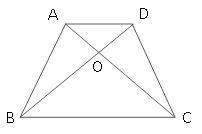### Sample Problem

In the trapezoid ABCD, shown here, if CO = 2AO, and the area of ∆DCO is 20 cm2, then what is the area of?

cm2#### SolutionWe see that ABC = BDC since they share the same base and height. We label (1) , (2) , (3) , and (4) , as shown. We know that (2) + (4) = (3) + (4) , so (2) = (3) . Since CO = 2AO, ADO and ODC have the same height, (2) = 2 (1) .

Also, since ABO and BOC have the same height, (4) = 2 (3) . The area of

(1) = 20 ÷ 2 = 10,

(3) = 20

and

(4) = 20 × 2 = 40.

Thus, the area of the trapezoid is

20 + 10 + 20 + 40 = 90 cm2.